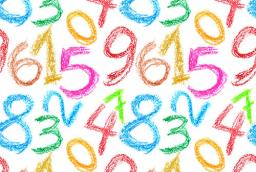# Subtraction 4809

I think the number when I multiply the imaginary number by three, we divide by the number 4 from the product of subtraction 4, and I add the imaginary number again to the proportion of 4. Which number do I think?

x =  7

### Step-by-step explanation:

(3x - 9)/4 +4 = x

(3·x - 9)/4 +4 = x

x = 7

x = 7/1 = 7

x = 7

Our simple equation calculator calculates it.Did you find an error or inaccuracy? Feel free to write us. Thank you!

Tips for related online calculators
Do you have a linear equation or system of equations and looking for its solution? Or do you have a quadratic equation?